# How to use the STDEV function in Excel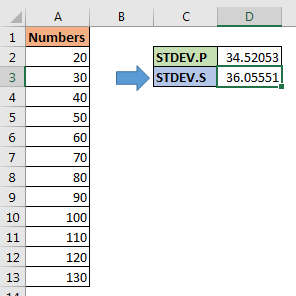In this article, we will learn How to use the STDEV function in Excel.

What is standard deviation and why is it used ?

Well, standard deviation is a calculated number that represents the dispersion of data from the mean or average of the dataset. It is calculated as the square root of variance. This is used to tell how far data spread from mean.

It is usually denoted as sigma.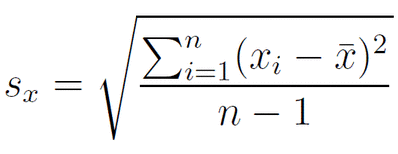Here

Sx is the standard deviation of the numbers

Xi is the numbers in the dataset

X with bar is called mean or average of numbers

n is the count of numbers in the dataset

Obviously Excel won't let you make this formula to calculate the standard deviation of the dataset. So it provides you with the inbuilt function named STDEV function. There are more versions of this function used for a different set of values.

STDEVP : This function used for data set having population

STDEV.S : This function used for data set have sample values

STDEV.P : This function is a newer version for STDEVP. Excel recommends this over STDEVP function.

STDEVA : This function accepts the text or cell reference values which other STDEV functions ignore.

STDEVPA : This function accepts population as data set and text and logic_values.

We recommend to use STDEV.S or STDEVS function in Excel

STDEV Function in Excel

Syntax :

 =STDEV (first_cell : last_cell)

 =STDEV (number1, [number2], ...)

number1, number2… can be given as array or individually using , as separator

Example :

All of these might be confusing to understand. Let's understand how to use the function using an example. Here we have a range of values from B1:B9 cell.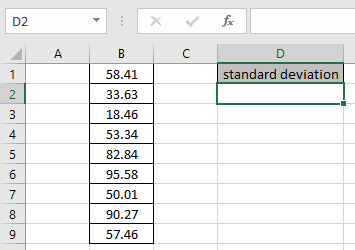We just need to use the formula

 =STDEV(B1:B9)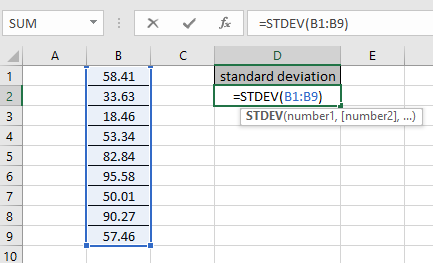Using the above formula in the cell to get the standard deviation of the values.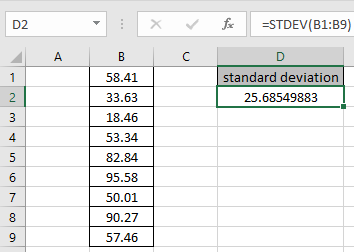The Standard deviation of the range is 25.685.

How to calculate STDEV manually in Excel and variation of STDEV.S and STDEV.P function

Excel provides two functions to calculate in standard deviation. STDEV.P and STDEV.S.

STDEV.P is used to calculate standard deviation, when you have captured whole population data.

When you have a sample of a large data, you should use the STDEV.S function. It is more accurate than standard deviation of population (STDEV.P).

If you calculate standard deviation on same data, using STDEV.S and STDEV.P. The STDEV.S will return a larger standard deviation. The standard deviation of a sample considers the chances of error and subtracts 1 denominator (number of observations). This is called Bessel’s Correction.

In above image, the standard deviation formula in D2 and D3 are:

 = STDEV.P(A2:A13) =STDEV.S(A2:A13)

How to calculate standard deviation manually?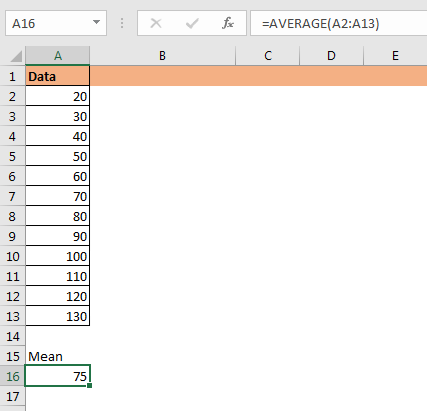Well as I stated in the beginning, standard deviation is square root of variance. And we have an excel function for calculating variance too. But we won’t use it. We will do it using the old school method.

Formula for standard deviation is

Standard Deviation ()= SQRT(Variance)

And Variance is

Variance = (sum of squared difference from mean) / number of observation

To calculate variance we need to calculate the difference of each number from mean of the data.

We get mean using AVERAGE function.

In cell A16 we have.

 =AVERAGE(A2:A13)

Now to calculate the squared difference from mean of each number, we write this formula in B2. copy down this formula.

 =POWER(Mean-A2,2)

Here the mean or average is in cell A16.

Now to get the sum of squared difference from mean, sum range B2:B13.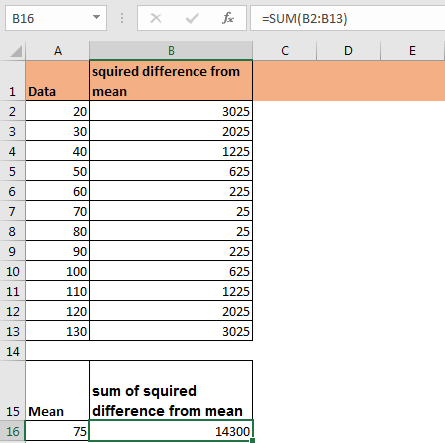To calculate variance of population, we just need to divide this sum of squared difference from mean by total number of observations. That is 12. Write this formula in C16 for variance of population.

 =B16/12             or =B16/COUNT(A2:A13)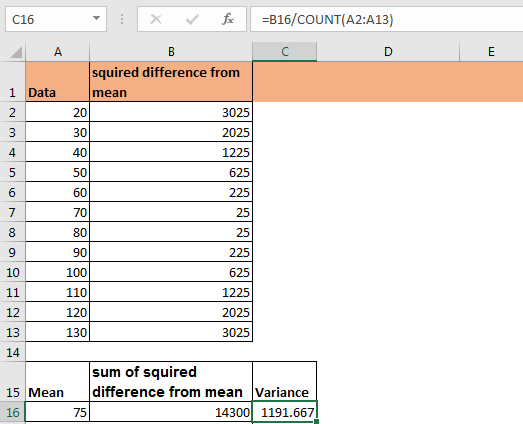This our variance of population. Which can be used to get Standard Deviation of Population (STDEV.P)  by getting square root of it.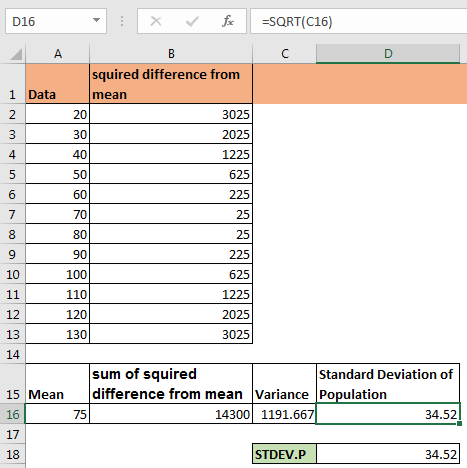To get standard deviation of sample we just need to subtract 1 from count of observations while calculating variance. This variance will variance of sample (VAR.P) and square root of it will be Standard Deviation of Sample (STDEV.S).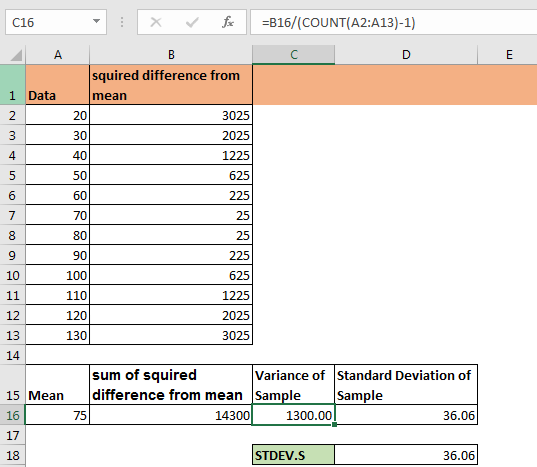The formula in C16 in above excel snapshot can be:

 =B16/11             or =B16/(COUNT(A2:A13)-1)

This is the manual calculation of standard deviation. Although, you don’t need to do manual calculation of standard deviation, it is good to know how the standard deviation is calculated in background.

There are two older functions for standard deviation in excel, STDEVS and STDEVP functions. They do the same thing as above standard deviation formulas. Don’t confuse between them. Excel recommends to use new STDEV.S and STDEV.P.

Hope this article about How to use the STDEV function in Excel is explanatory. Find more articles on calculating values and related Excel formulas here. If you liked our blogs, share it with your friends on Facebook. And also you can follow us on Twitter and Facebook. We would love to hear from you, do let us know how we can improve, complement or innovate our work and make it better for you. Write to us at info@exceltip.com.

Related Articles :

How to Calculate Average or Mean in Excel : Find the average or of the numbers (given as array) using the AVERAGE function in Excel. In Mathematics average and mean are same in functionality.

How to use the VAR function in Excel : Calculate the variance for the sample dataset in excel using the VAR function in Excel.

How to Calculate Standard Deviation in Excel : To calculate the standard deviation we have different functions in Excel. The standard deviation is the square root of the variance value but It tells more about the dataset than variance.

Regressions Analysis in Excel : Regression is an Analysis Tool, which we use for analyzing large amounts of data and making forecasts and predictions in Microsoft Excel.

How to Create Standard Deviation Graph : The standard deviation tells how much data is clustered around the average of the data. Learn how to create a standard deviation graph here.

How to use Excel NORMDIST Function : Calculate the Z score for the normal cumulative distribution for the pre specified values using the NORMDIST function in Excel.

How to use Excel NORM.INV Function : Calculate the inverse of Z score for the normal cumulative distribution for the pre-specified probability values using the NORM.INV function in Excel.

Popular Articles :

How to use the IF Function in Excel : The IF statement in Excel checks the condition and returns a specific value if the condition is TRUE or returns another specific value if FALSE.

How to use the VLOOKUP Function in Excel : This is one of the most used and popular functions of excel that is used to lookup value from different ranges and sheets.

How to use the SUMIF Function in Excel : This is another dashboard essential function. This helps you sum up values on specific conditions.

How to use the COUNTIF Function in Excel : Count values with conditions using this amazing function. You don't need to filter your data to count specific values. Countif function is essential to prepare your dashboard.

Terms and Conditions of use

The applications/code on this site are distributed as is and without warranties or liability. In no event shall the owner of the copyrights, or the authors of the applications/code be liable for any loss of profit, any problems or any damage resulting from the use or evaluation of the applications/code.